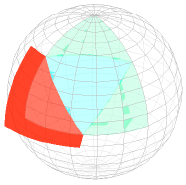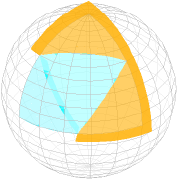## SOHO: Orthogonal and Symmetric Haar Wavelets on the SphereFigure 1: Our derivation yields two bases that are orthogonal and symmetric. The two sets of basis functions for a partition at level 0 are shown above.

Abstract: We propose the SOHO wavelet basis. To our knowledge this is the first spherical Haar wavelet basis that is both orthogonal and symmetric, making it particularly well suited for the approximation and processing of all-frequency signals on the sphere. The key to the derivation of the basis is a novel spherical subdivision scheme that defines a partition acting as domain of the basis functions. The construction of the SOHO wavelets refutes earlier claims doubting the existence of such a basis. We also investigate how signals represented in our new basis can be rotated. Experimental results for the representation of spherical signals verify that the superior theoretical properties of the SOHO wavelet basis are also relevant in practice.

Publications

Christian Lessig and Eugene Fiume, SOHO: Orthogonal and Symmetric Haar Wavelets on the Sphere, ACM Transactions of Graphics, March 2008 (Presented at SIGGRAPH 2008). (preprint, bibtex)

Christian Lessig, Orthogonal and Symmetric Haar Wavelets on the Sphere, Master of Science thesis, Department of Computer Science, University of Toronto, April 2007. (pdf, bibtex)

Miscellaneous

Matlab implementation of the SOHO wavelets.

Presentation slides from SIGGRAPH 2008.

Comparison of Spherical Harmonics and the SOHO wavelets.

Video detailing the construction of the SOHO wavelets.

Presentation slides from my MSc. defense.

Mathematica notebook detailing the construction of the basis functions of the SOHO wavelets.

Mathematica notebook detailing the construction of the subdivision scheme for the partition of the SOHO wavelets.

A short write-up on second generation wavelets (used as hand-out for a blackboard-based talk).

 © lessig (at) dgp (dot) toronto (dot) edu August 2008